Dependence of Rate of Reaction in CHEMICAL KINETICS, an innovative topic of Physical Chemistry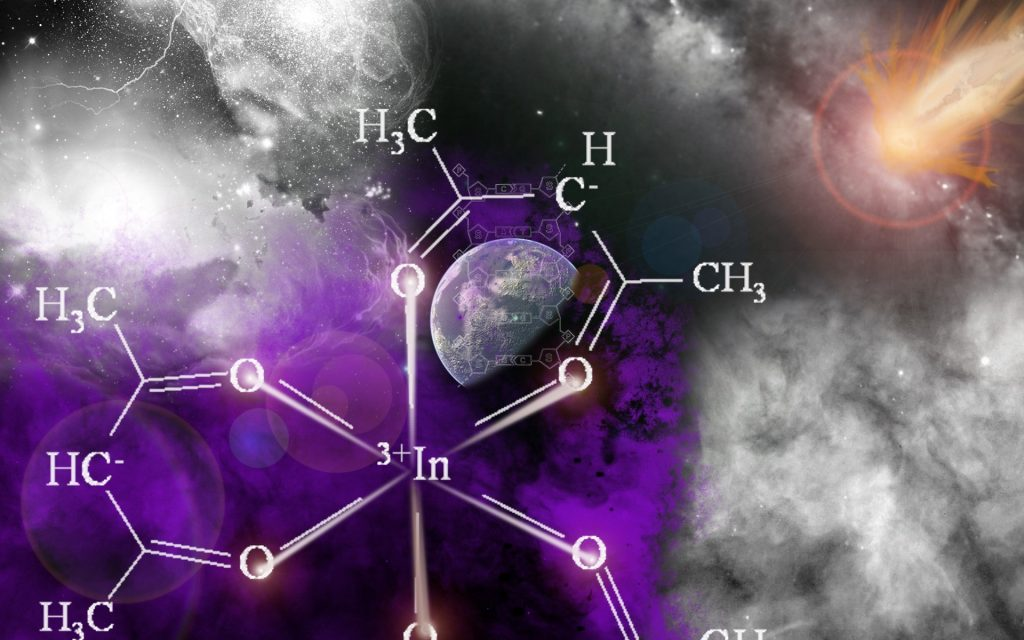Dependence of Reaction Rates on concentration (Rate law expression)

When a chemical reaction proceeds, concentration of reactant decreases while that of products increases.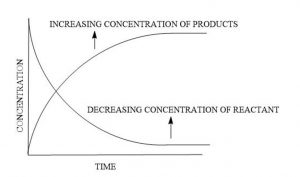→ It is indicated from the fig, In the biginning, only reactants are present so that the concentration of product is Zero
→As the reaction proceeds, the concentration of the reactants decreases and that of preoduct increases.
→At the start of the reactant, rate of reaction is large and it decrease with passage of time. It means that rate of reactant is directly proportional to concentration of reactants. To increase the rate of a reactant we should increase the concentration of one or all reactants and vice.versa.
→In the gas phase, the increse in pressure of the reactant gases increase in pressure, the number of molecule per unit valume increase and therefore, rate of reactant increase.

Law of Mass Action

→The quantitave relationship between the rate of a reaction and the molar concentration of the reacting substance was given by Norwegian Chemists, Guldbege and waage. This relationship is called Law of mass action
→According to his law; At a given temperature the rate of chemical reactant is directly proportional to the product of the molar concentration of the reactants. The molar concentration of reactants is also called active mass.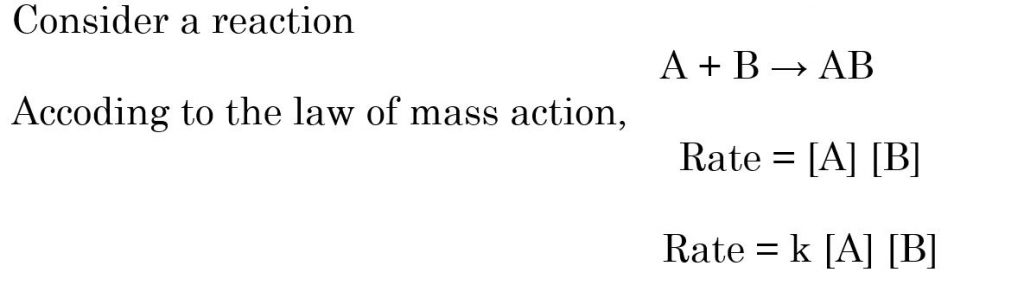where [A] and [B] are molar concentration of [A] and [B]
K → Proportionality constant, called rate contant, also called velocity constant
If [A] = [B] = 1 (unity) then rate = K
Thus rate constant of a reactnat at given temperture may be defined a rate of reaction when concentration of each of the reactant is taken as unity

Characteristic of a Rate constant

The rate constant has the following properties-
(i) Rate constant is a measure of rate of reaction
Larger the value of K , Faster is the raection
Smaller the value of K , Slower is the reaction
(ii) Different reactnt have different value of K
(iii) At a fixed temperture, the value of K constant and is characteristic of the reaction. However, it changes with temperature
For the particular order reactant, the rate constant is independent of concentration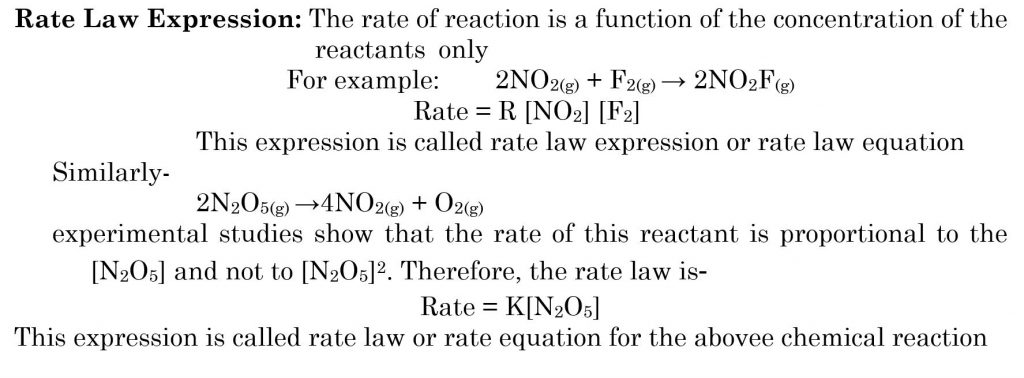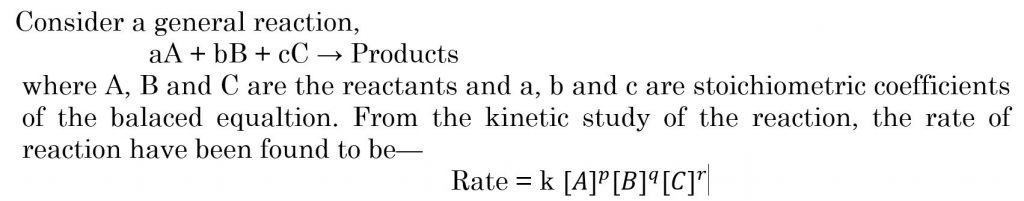where p, q, and r are constant numbers or the power of the concentration of the reactant A, B and C respectively, on which the rate of reactant depends
—The values p, q and r are determined experimentally and may or may not be equal to a, b and c
—The mathemical expression which denotes the experimentally observed rate of a reaction in terms of the concerntrations of the reacting species which influences the rate of reactant is called rate law or rate equation.
— The power of concentration of reactnts in rate law expession may or may not be same as the coeefucient of reactant in the balanced chemical equation.
— On the other hand., the law of mass action given the rate expression on the basis of overall balanced equation.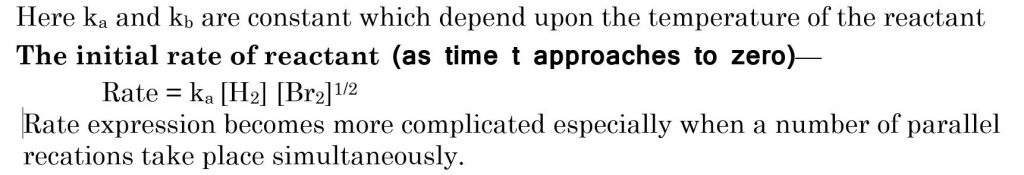All the topics studied under Chemical Kinetics are very useful for CSIR NET coaching in Chemistry, GATE coaching in Chemistry and IIT JAM coaching in Chemistry.

CHEMICAL KINETICS – An Essential Branch of Chemistry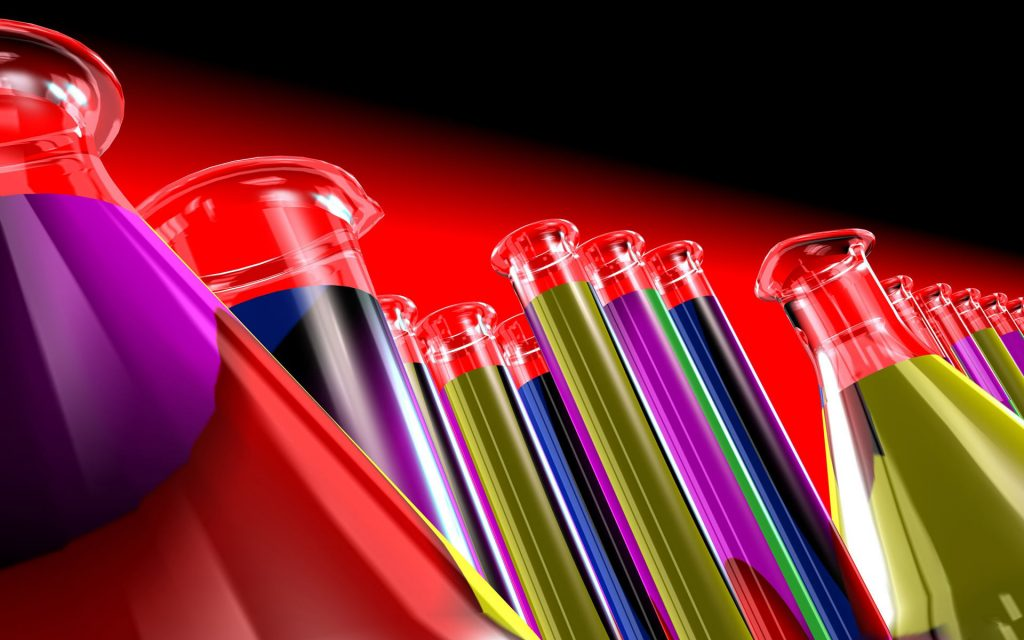The feasibility of a given reaction can be predicted with the help of thermodynamic principles. Besides this, the relative amounts of reactants and products at equilibrium position of the reaction can also be predicted. In addition, we can predict whether changes in the experimental conditions will increase or decrease the amount of a product at equilibrium. However, thermodynamic principles do not provide any information regarding the speed of the given reaction, i.e. how much time a given feasible reaction will take for its completion. Moreover, it is not possible to predict how the speed of a given reaction gets affected by changing the experimental conditions. The study of rate of reactions including its dependence on the concentrations of reacting species and the experimental conditions constitutes one of the topics of physical chemistry and is known as chemical kinetics. The important application of the study of rate of reaction is to use the kinetic data in establishing the molecular pathway or mechanism by which the reaction takes places. This branch of chemistry is very useful for CSIR NET coaching in Chemistry, GATE coaching in Chemistry and IIT JAM coaching in Chemistry.

Rate of Reaction

The rate of a chemical reaction is a measure of the speed with which the reactants are converted into products. It may be defined as the change in any one of the reactants or products per unit time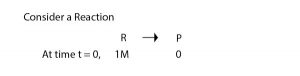In this reaction, in unit volume, one mole of the reactant (‘R’) produces one mole of the product ‘P’. The rate of reaction may be expressed by the following two ways ______
(i) The rate of disappeorance or decrease in concentration of R (reactant)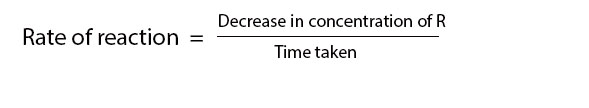(ii) The rate of increase in concentration of P (products)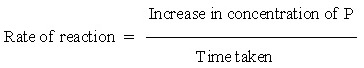If [R1] and [P1] are concentration of R & P at time t1 and [R2] and [P2] are concentration at time t2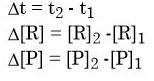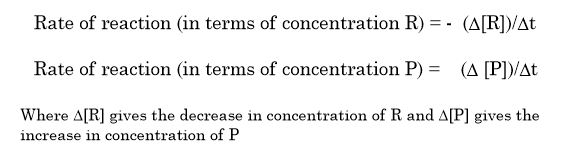Where ∆[R] gives the decrease in concentration of R and ∆[P] gives the increase in concentration of P
The square brackets arround the substances are used to express the molar concentration (mol/litre or mole litre-1)
→ In case of concentration of reactants, we use minus sign
This implies that the concentration of reactants of decreasing with time
∆[R] → negative value
rate always → positive so
we inlude minus sign in the expression of rate with respect to reactant
The above rate is called average rate of the reaction. It has the dimensions of concentration/time. So the unit of rate of reaction will be concentration time-1
if unit of concentration is → mole litre-1 (Molarity)
and unit of time → second
then unit of rate of reaction will be → Mole litre-1 s-1
→ However in gaseous reactions, when concentration of gases is expressed in terms of their partial pressure, then unit of rate of reaction → atmosphere s-1
→ In the above example the stoichimetric coefficients for the reactants and products are same

A + B → 2C

In this case, one mole of A reacts with one mole of B to form 2 moles of C. This means that the rate of disappearance of A and B are same but the rate of disappearance of C is the twice the rate of disappearance of A and B. Thus,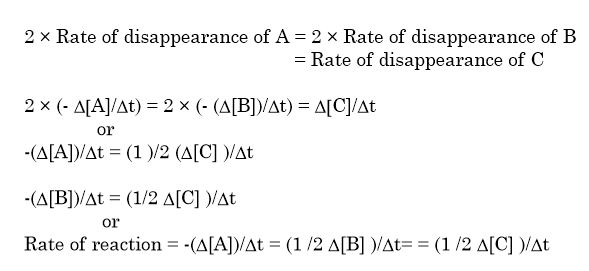e.g. For the decomposition of gaseous nitrogen pentaoxide (N2O5) as –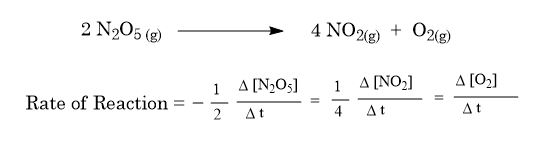Average Rate and Instantantaneous Rate
→ The rate expressions given the average rate of reactions over the time interval (∆t). This is expressed as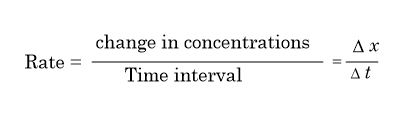→ This concept is similar to mechanical speed.
→ In case of reactions the rate depends upon the concentration of the reactants. As the reaction proceeds, the concentration of the reactants decreases with time. Thus, the rate of reaction may not be constant in the time interval which we measure. Thus, the rate cannot be determined simply by dividing the total change in concentration by the time taken as in case of mechanical speed.
→ On the other hand, rate of reaction may be expressed at a particular moment of time thus, rate of reaction may be defined as the rate of change of concentration of any one of the reactants or products at a given time. Such a reaction rate is also known as instantaneous rate.
→ Mathematically, instantaneous rate may be represented by-(d[R])/dt or (d[P])/dt instead of – (∆[R])/∆t or (∆[P])/∆t respectively. Here d[R] or d[P] represents infinitesionally small changes in concentration of R or P in infinitesimally small interval of time, dt. Thus average rate approches the instantanious rate as ∆t becomes smaller and smaller i.e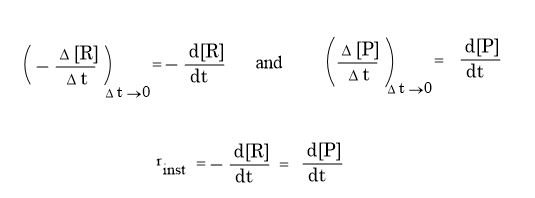In general, if dx represents very small (infinitesimally small) change in concentration o any species during the very small (infinitesimally small) change of time, at the rate of reaction can be expressed as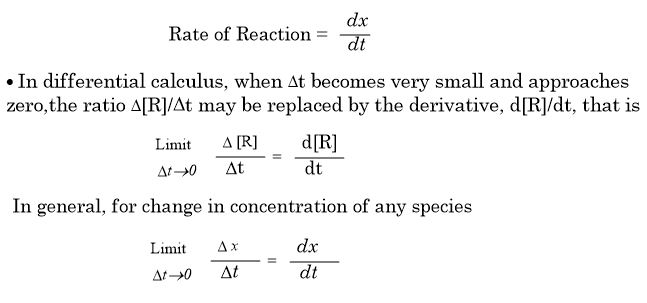where dx is the infinitesimally small change in concentration corresponding to small change in time, dt.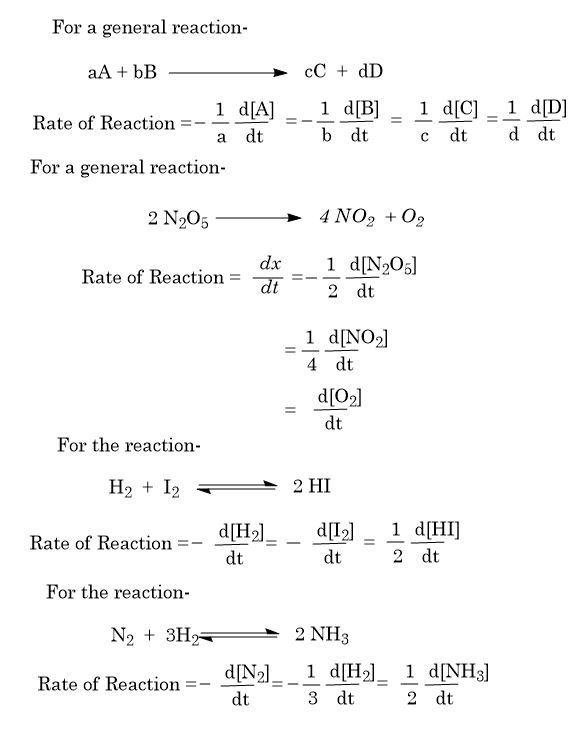All the topics studied under Chemical Kinetics are very useful for CSIR NET coaching in Chemistry, GATE coaching in Chemistry and IIT JAM coaching in Chemistry